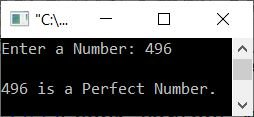# C++ program to check the perfect number

This page is created to provide some programs in C++ that are used to check whether a number entered by the user is a perfect number or not. But before going through all these programs, which are:

Let's first understand what perfect numbers are.

#### Which number can be called a perfect number?

If a number is equal to the sum of its positive divisors (excluding the number itself), then that number can be called a "perfect number." For example, 28 is a perfect number because the divisors of 28 in this case are 1, 2, 4, 7, and 14. And the sum of all these numbers gives the number itself. That is:

```28 = 1+2+4+7+14
= 14+14
= 28```

Therefore, 28 is a perfect number. Some other perfect numbers are 6, 496, 8128, etc. Now let's move on to the program given below.

## Using the for loop, determine the perfect number

The question is: write a program in C++ that checks whether a number is a perfect number or not. The user must enter the number during program execution. The answer to this question is:

```#include<iostream>

using namespace std;
int main()
{
int num, i, sum=0;
cout<<"Enter a Number: ";
cin>>num;
for(i=1; i<num; i++)
{
if(num%i==0)
sum = sum+i;
}
if(num==sum)
cout<<endl<<num<<" is a Perfect Number.";
else
cout<<endl<<num<<" is not a Perfect Number.";
cout<<endl;
return 0;
}```

Here is its initial output:Now enter a number as input, say 496, and press the ENTER key to check whether it is a perfect number or not, as shown in the snapshot given below:The dry run of the above programme with user input 6 goes like this:

• When the user enters a number, it gets stored in the num variable. Therefore num=6
• Now the execution of the for loop begins, starts with i's value of 1.
• With i = 1, the condition i < num or 1 < 6 evaluates to be True, therefore program flow goes inside the loop.
• The condition (of if) num%i==0 or 6%1==0 or 0==0 evaluates to be True, therefore program flow goes inside the if block or in the body of if.
• and sum+i or 0+1 or 1 gets initialized to sum. Therefore, sum=1
• Now the value of i gets incremented using the i++ statement. As a result, i=2 now.
• Again, the condition i<num or 2<6 evaluates to be True, therefore program flow again goes inside the loop
• And inside the loop, again the condition (of if), num%i==0 or 6%2==0 or 0==0 evaluates to be True
• Therefore sum+i or 1+2 or 3 gets initialized to sum. Now sum=3
• Now the value of i again gets incremented using i++. Now i=3
• And the condition i<num is evaluated again with the new value of i.
• This process continues, until the condition of the loop is evaluated as false.
• In this way, after exiting from the loop, the sum variable holds the sum of all the divisors of the number.
• As a result, I compared the sum to the number itself. If it is equal, then it is a perfect number. Otherwise not

## Check the perfect number using the while loop

To change the above program and create a new program using the while loop, follow the steps given below:

• initialize i with 1 at the time of its declaration.
• put the condition i<num inside the while loop as it is.
• increment the value of i in the body of the loop, like shown in the program given below.

Here is the program that uses the while loop instead of the for loop to do the same job as the previous program.

```#include<iostream>

using namespace std;
int main()
{
int num, i=1, sum=0;
cout<<"Enter a Number: ";
cin>>num;
while(i<num)
{
if(num%i==0)
sum = sum+i;
i++;
}
if(num==sum)
cout<<endl<<num<<" is a Perfect Number.";
else
cout<<endl<<num<<" is not a Perfect Number.";
cout<<endl;
return 0;
}```

This program produces the same output as the previous program.

## Check the perfect number using the function

This is the last program of this article, created using a user-defined function named check_per(). This function returns 1 if the number is a perfect number; otherwise, it returns 0.

```#include<iostream>

using namespace std;

int check_per(int);
int main()
{
int num, res;
cout<<"Enter a Number: ";
cin>>num;
res = check_per(num);
if(res==1)
cout<<endl<<num<<" is a Perfect Number.";
else
cout<<endl<<num<<" is not a Perfect Number.";
cout<<endl;
return 0;
}
int check_per(int n)
{
int i, s=0;
for(i=1; i<n; i++)
{
if(n%i==0)
s = s+i;
}
if(n==s)
return 1;
else
return 0;
}```

This program again does the same job as the previous program and produces the same output.

C++ Quiz

« Previous Program Next Program »